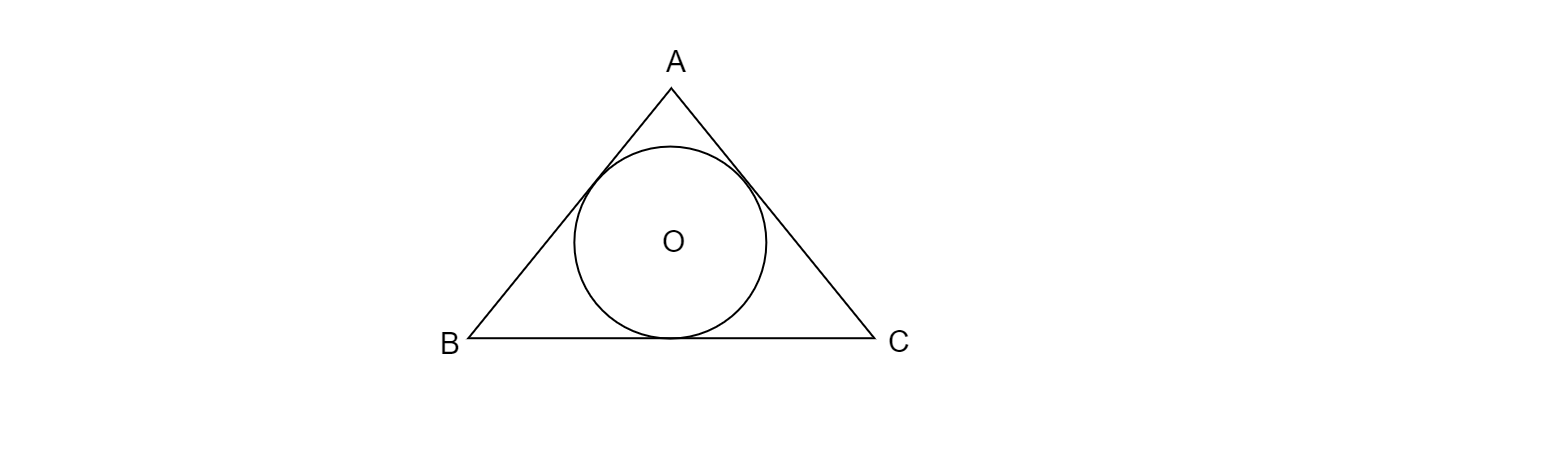"
">

# In Fig 4, a circle is inscribed in an equilateral triangle $\vartriangle ABC$ of side $12\ cm$. Find the radius of inscribed circle and the area of the shaded region. [$Use\ \pi =3.14\ and\ \sqrt{3} =1.73$]."

Given: Fig 4, an equilateral triangle ABC of side 12 cm, and a circle inscribed in the given triangle.

To do: To find the radius of inscribed circle and the area of the shaded region.

Solution:It is given that $\vartriangle ABC$ is an equilateral triangle of side 12 cm.

$\therefore AB=BC=CA$

Join $OA,\ OB$ and $OC$

Draw $OP \perp BC$

$OQ\perp AC$

$OR \perp AB$

Let the radius of the circle be $r\ cm$.

$OR=OP=OQ=r$

In $\vartriangle AOB$,

AB is the base  and OR is the height of the $\vartriangle AOB$,

$\therefore$ Area of $\vartriangle AOB$,$A_{1} =\frac{1}{2} \times base\times height$

$\Rightarrow A_{1} =\frac{1}{2} \times AB\times r$

$\Rightarrow A_{1} =\frac{1}{2} \times 12\times r$

$\Rightarrow A_{1} =6r$

similarly areas of $\vartriangle AOC$ and $\vartriangle BOC$ are:

$A_{2} =6r$

$A_{3} =6r$

Area of $\vartriangle ABC=\frac{1}{2} \times sin\theta \times ( side)^{2}$

$=\frac{1}{2} \times sin60^{o}\times 12\times 12$

$=\frac{1}{2} \times \frac{\sqrt{3}}{2} \times 144$

$=36\sqrt{3} \ cm^{2}$

Area of $\vartriangle ABC=$Area of $\vartriangle AOB+$Area of $\vartriangle AOC+$Area of $\vartriangle BOC$

$\Rightarrow 6r+6r+6r=36\sqrt{3}$

$\Rightarrow 18r=36\sqrt{3}$

$\Rightarrow r=\frac{36\sqrt{3}}{18}$

$\Rightarrow r=2\sqrt{3} \ cm$

Area of the circle$=\pi r^{2}$

$=\pi( 2\sqrt{3})^{2}$

$=12\pi \ cm^{2}$

Area of the shaded region$=$Area of $\vartriangle ABC-$Area of the circle

$=\ 36\sqrt{3} -12\pi$

$=( 36\times 1.73-12\times 3.14)$

$=( 62.28-37.68)$

$=24.6\ cm^{2}$

Therefore, The area of the shaded region is $24.6\ cm^{2}$ .

Updated on: 10-Oct-2022

101 Views